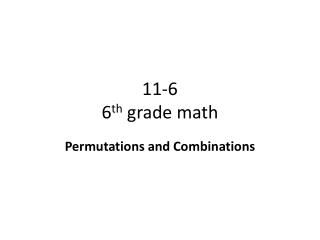# 11-6 6 th grade math - PowerPoint PPT PresentationDownload PresentationDownload Presentation## 11-6 6 th grade math

- - - - - - - - - - - - - - - - - - - - - - - - - - - E N D - - - - - - - - - - - - - - - - - - - - - - - - - - -
##### Presentation Transcript

1. 11-66th grade math Permutations and Combinations

2. Objective • To count the number of ways to choose things when order does and does not matter • Why? To know how to count arrangements when order matters (permutations)and when order does not matter (combinations).

3. California State Standards SDP 3.1 : Represent all possible outcomes of compound events in an organized way (e.g., … grids, tree diagram) … MR 2.4: Use a variety of methods, such as words, numbers, symbols, charts, graphs, tables, diagrams, and models, to explain mathematical reasoning.

4. Vocabulary • Permutation • Each possible arrangement of the outcomes of an event where order is important. Will be a bigger number than a combination. • To solve: multiply using factorial product of how many ways. The answer is the number of possibilities. The factorial product = x! • Who will be president and who will be treasurer • 23 students; (23 students, – 1 other student = 22) • 23 x 22 = 506 permutations To remember is permutation: Think of a perm in your hair. Ordering the way the hairdresser puts a perm in your hair is very important. Combination • Each possible arrangement of the outcomes of an event where order is not important. Will be a smaller number than a permutation. • To solve: permutation ÷ number in the combo • Two people wanting to be president/treasurer • 506/2 = 253 combinations • Ways to choose a class treasurer after president is chosen Think of the ways you can comb your hair. The order in which your comb your hair really does not matter.

5. How to Solve Permutations 1) Read problem. Be sure order does matter (permutation). 2) Multiply the factorial product by number of arrangements needed. 3) Multiply carefully Put 2 different-colored balloons on display. 5 Colors: red, blue, yellow, green, orange. How many different arrangements? Order matters, don’t repeat same 2 colors. 5! by 2 spaces 5 x 4 = 20 different arrangements

6. How to Solve Combinations 1) Read problem. Be sure order does NOT matter (= combination). 2) Divide the permutation by the number in the combo 3) Divide carefully Find the number of arrangements to make if put in 2 different colored balloons and order of arrangement does not matter. Permutation = 5 x 4 = 20 # in combo = 2 colors 20/2 = 10 choices or arrangements

7. Try It! #1 & 2) Decide whether or not order matters in each situation. • Choosing 5 CD’s from a list of 20 • Choosing 5 digits for a password • How many 3-letter permutations can be made from the letters GREAT? • 4 kinds of fruit. Put 3 kinds in a basket. How many specific arrangements (permutations)? • Order not matter • Order does matter • 5! By 3 spaces = 5 x 4 x 3 = 60 permutations 4) 4! By 3 spaces = 4 x 3 x 2 = 24 arrangements

8. Objective Review • To count the number of ways to choose things when order does and does not matter • Why? You now know how to count arrangements when order matters (permutations)and when order does not matter (combinations).

9. Independent Practice • Complete problems 5-11 • Copy original problem first. • Show all work! • If time, complete Mixed Review: 12-15 • If still more time, work on Accelerated Math.